## Thursday, March 31, 2011

### Fitting Oriented Bounding Boxes

It can be useful to fit oriented bounding boxes (OBBs) to sets of geometric primitives for a number of applications. This post describes one way to do this, based on the PhD thesis of Stefan Gottschalk. I have put together a sample implementation that you can use and adapt as you wish.

The method of fitting is based around the covariance matrix of the items to be fit. The covariance matrix is a generalization of variance to higher dimensions. The use of the covariance matrix in fitting an oriented bounding box is that if the covariance matrix is constructed carefully, its eigenvectors determine the rotation required to obtain a tightly fitting box.

The overall process for fitting a bounding box will then be:
• Compute the mean position and covariance matrix of the inputs
• Extract the eigenvectors of the covariance matrix
• Build a transformation matrix using the mean position and eigenvectors
• Size the box to fit the input

Given a decent linear algebra library (such as gmm++), only the first task is particularly complicated, so I'll discuss it first.

In the case of fitting a bounding box to a collections of objects, there are three dimensions X, Y and Z, so the covariance matrix is 3x3. The covariance matrix for this case is shown below: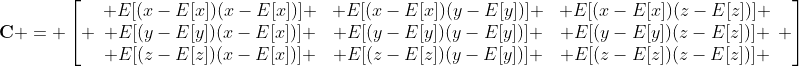In the above equation x, y and z are the three global coordinates, and E[arg] is the expected value of the argument arg, which in this case is the mean of arg. If the mean values of x, y and z are denoted by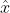,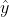and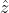,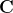can be written as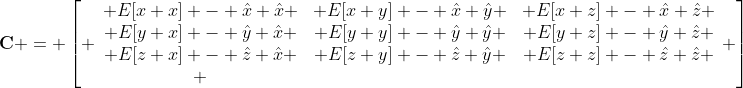where the simplification is due to the properties of the expected value. Armed with this definition, we can begin computing covariance matrices for sets of points. This is the simplest case, and can produce decent bounding boxes if the points are well distributed.

We start by computing the mean positions:where N is the number of points in the point set. This leaves us needing to compute the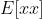,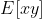, ...,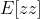terms. Since we are dealing with points, these turn out to be very simple: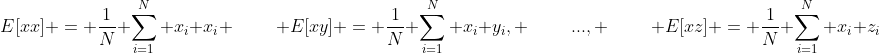and the analogous expressions for the other terms. With these expressions, we can now build the covariance matrixand extract its eigenvectors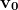,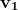and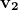using our math library. Each of the three eigenvectors will be a three-component vector, and will be orthogonal to the other two eigenvectors sinceis symmetric.

To build the OBB transformation matrix we compute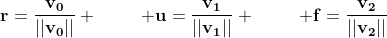and then use them as the columns of the rotation portion of the OBB transformation matrix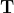, i.e.: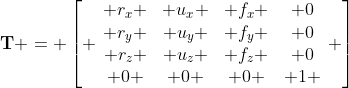All that remains is to figure out how big to make the box and where to put it so that it contains all the points. To do this, we transform each point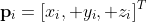into the local frame of the box using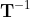and keep track of the minimum and maximum coordinates in each direction. Since the rotation part of the matrix is orthogonal and the translation part is zero, a shortcut for transforming the points without having to invertis as follows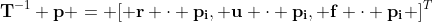whereis the vector dot-product and everything else is defined as before. We then find the minimum and maximum of each coordinate of the transformed points for each coordinate, storing them in the 3D points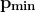and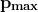. The half-extents of the bounding box will then beand the center position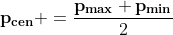, both in the local frame of the box. The corresponding world-space coordinate of the centercan be found by multiplying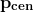by. Oncehas been computed, it can be added into the transformation matrix to get the final transformation matrix for the OBB: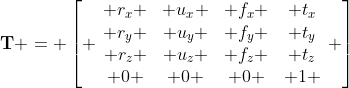The result is an OBB that contains the input. I have used the Stanford bunny below as a sample model:

Unfortunately, if the points are not well distributed the box may not be a tight fit. The reason for this is that variations in point sampling density can skew the eigenvectors of the covariance matrix and result in a poor orientation being found.

One way to make this process more robust so that tighter bounding boxes can be found is to build the covariance matrix using the triangles of the model rather than the vertices, and integrate over the surface area of the model rather than simply summing the contributions tofrom each vertex.

In this case, the formulas for the terms needed to buildare slightly more complicated than for points:where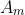is the surface area of the entire model,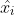,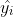andare the coordinates of the centroid of triangle i and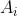is the area of triangle i. The remaining terms,(and analogous), are also a bit more complicated: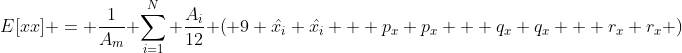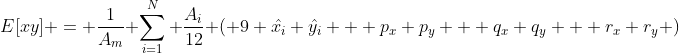where p, q and r are the vertices of triangle i. The remaining expressions for,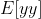,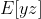andare analogous, and can be found by replacing x and y with the appropriate variables in the above expressions. With these expressions for the covariance matrix of the model, we can then build an OBB in the same manner as before. In most cases, this change will be enough to obtain a tight box. However, if the model has large interior features, these can again mess up the eigenvectors of the covariance matrix and result in a box that doesn't fit the object tightly.

Using triangles instead of points gives a slightly tighter result for the Stanford bunny:There is one final improvement that can be made which will result in a method that is robust for all inputs. Instead of building the covariance matrix of the input itself we instead build the covariance matrix of the convex hull of the input. The convex hull approximates the shape of the input, and in the process removes interior surfaces that cause errors in fitting tight boxes. Computing the convex hull of a 3D object is somewhat involved, however there are libraries that do it which can be used (for example CGAL). Note however that the convex hull may well have a very non-uniform point distribution, so the triangle-based covariance matrix should be used and not the point-based version.

The convex hull version gives an even tighter box than the point version, but is substantially slower in my implementation (and should be in general) due to the complexity of building the convex hull of the input. Even though I have isotropically remeshed the bunny, there are still significant differences in the OBB orientations for the three methods, even though the point sampling and triangle sizes are relatively uniform.OBB fit using pointsOBB fit using trianglesOBB fit using convex hull

I have put together a sample implementation of the three methods of fitting OBBs discussed in this posting. It depends on CGAL for computing convex hulls and gmm++ for linear algebra. Feel free to use it for whatever you'd like.